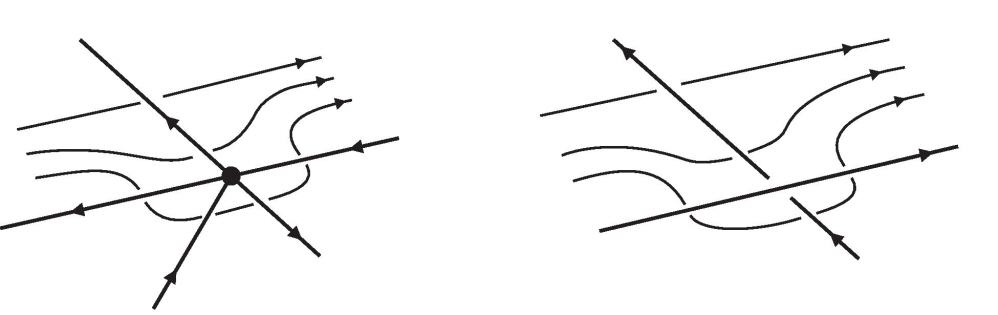# Obtaining Wirtinger presentation using van Kampen theorem

Hatcher's Algebraic Topology, section 1.2, problem #22 describes an algorithm for computing the Wirtinger presentation of the complement of a smooth or piecewise linear knot K in $\mathbb{R}^3$:

Position the knot to lie almost flat on a table, so that K consists of finitely many disjoint arcs $\alpha_i$ where it intersects the table top together with finitely many disjoint arcs $\beta_l$ where K crosses over itself...We build a 2-dimensional complex X that is a deformation retract of $\mathbb{R}^3-K$ by the following three steps. First, start with the rectangle T formed by the table top. Next, just above each arc $\alpha_i$ place a long, thin rectangular strip $R_i$, curved to run parallel to $\alpha_i$ along the full length of $\alpha_i$ and arched so that the two long edges of $R_i$ are identified with points of T...Any arcs $B_l$ that cross over $\alpha_i$ are positioned to lie in $R_i$. Finally, over each arc $B_l$ put a square $S_l$ bent downward along its four edges so that these edges are identified with points of three strips $R_i$, $R_j$, and $R_k$...namely, two opposite edges of $S_l$ are identified with two arcs crossing the interior of $R_i$. The knot K is now a subspace of X, but after we lift K up slightly into the complement of X, it becomes evident that X is a deformation retract of $\mathbb{R}^3-K$.

The actual problem is to assume this geometry is valid, and then "apply van Kampen's theorem at each step" to get the Wirtinger presentation. My main trouble with the problem is trying to actually make sense of this geometry.

First, if the knot is "almost flat" on the table, does that mean that it is entirely on top of the table, in such a way that if I think of the string as a road, cars going across it will have to take some kind of almost-u-turn in the transition from one of the $\alpha_i$ arcs to another? I'm struggling to describe what I'm talking about, but it's the only way I can make physical sense of the entire knot being on the table, but also divided into multiple different pieces ("arcs").

Next, the squares. The best I can tell from the diagram is that the square "$S_l$" is really just a piece cut out of $R_i$ with the two bottom (i.e., table-intersecting) edges that touch $R_j$ and $R_k$ identified with the bottom pieces of those strips at the ends where they meet it. Is that right, or way off base?

Finally, the last sentence. I can't imagine how just picking K up off of X shows that it is a deformation retract of the entire knot complement. I don't think a more detailed explanation of this is actually necessary for proving the Wirtinger presentation of the fundamental group, since the problem basically allows me to assume this is true and it isn't actually one of the steps for "constructing" X, but I'd still like to have some understanding of it. Any help with seeing what this retraction looks like would be appreciated.

Ok, let me answer these questions in the order you asked them. About almost flat, I could not understand the 'U' turns part of your question, but to see what Hatcher means by almost flat, you should think about this picture:So, the only time our knot is not in the $x,y$ plane is at the crossing and the overarc just is bumped up (in the positive $z$ direction) slightly so it doesn't intersect the underarc. The arcs $\alpha_i$ are the pieces of the knot that are in the $x,y$ plane. In our picture, that is everything except the small bump in the red arc. The $\beta_l$'s are the bumps that come out of the plane. So, the whole knot is the union of all the $\alpha_i$'s and $\beta_l$'s.

Next, the square $S_l$ is not a subset of $R_i$. Here is a picture of the $R_i$'s before we add in $S_l$.I would think of $S_l$ as continuing $R_j$ but it rides over $R_i$, so two edges of its boundary lies in $R_i$ but nothing else.Last, now that we have $X$, we can think of "picking up $K$" as literally just shifting the knot up in the $z$ direction slightly, so that it is in the center of the space between the table top and $R_i$'s. So, in our picture, the knot is the red and blue arc, which used to lie in the green table, but we move it so it is in the center of the space we made with the $R_i$'s and $S_l$'s.To imagine the retract, it is the same idea as taking $\mathbb{R}\setminus \{x$-axis$\}$ and retracting to an infinite cylinder around the $x$-axis. For us, instead of the $x$-axis, we have a loop, so instead of a cylinder, we would get a torus, but the crossings make us identify parts of the torus. Also, we get the table top as part of our retract.

• Ah, ok. The $\alpha_i$'s are the pieces of the knot between "bumps." So, in your picture, $a_2$ and $a_3$ are should be the same, both $a_2$, since they are not seperated by bump. Sep 13 '14 at 14:51